Editor Ratings:
User Ratings:
[Total: 0 Average: 0]

Here are 5 free Kinetic Energy calculator websites. Using these websites, you can calculate Kinetic Energy of various objects. Many of these websites let you choose various units from Kinetic Energy Parameters like mass and velocity. Not only Kinetic Energy but these websites can also calculate mass, velocity if you provide the energy value. All of these websites come with an intuitive interface and you can easily calculate Kinetic Energy with them.

Although, you can calculate Kinetic Energy using many scientific calculators. But if you want to specifically calculate Kinetic Energy online, then these websites present a good option.## Kinetic Energy Equations Formulas Calculator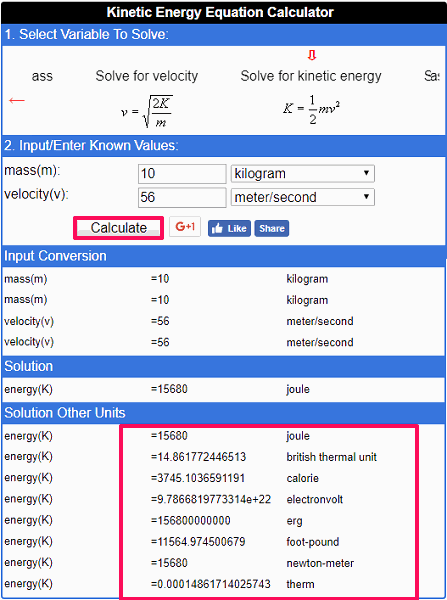Kinetic Energy Equations Formulas Calculator is one of the best free Kinetic Energy Calculator websites that you can use. This website lets you calculate Kinetic energy in various units. Also, you will see the calculated Kinetic Energy in all the units that it supports. It supports Joule, British Thermal Units, Calorie, Electron volt, etc. units to denote the Kinetic energy.

Using this website you can easily calculate the Kinetic Energy in just a few seconds. Open the homepage of the website and then specify the mass and velocity in the respective units you want. Finally, hit the Calculate button and it will calculate the Kinetic Energy for you. And you will see that It shows the Kinetic Energy in various units as I have shown in the above screenshot.

## Kinetic Energy Calculator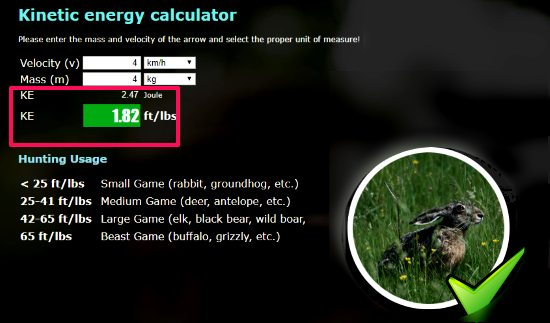Kinetic Energy Calculator (homepage) is another very good website to calculate Kinetic Energy. This is a dedicated website to calculate Kinetic Energy and after specifying the mass and velocity, it will give you the result. It supports 3 different units for mass, velocity, and Kinetic Energy. There is one unique thing on this website: To demonstrate the Kinetic Energy, it shows different picture of animals. For example, if you specify that the Kinetic Energy is less than 25 ft/lbs, then it will denote it with a rabbit. And if the Kinetic Energy value is greater than 65 ft/lbs, then it will show you the picture of buffalo or some other animal.

Using this free website to calculate Kinetic Energy is pretty simple. Just visit the website and then specify the values for mass and velocity. After that, it will automatically calculate the Kinetic Energy for you. It places the result along with the conversion of the Kinetic Energy value in ft/lbs. Also, you will see a picture of an animal which has the similar Kinetic Energy on its interface. You can see it in the above screenshot.

## Calculator City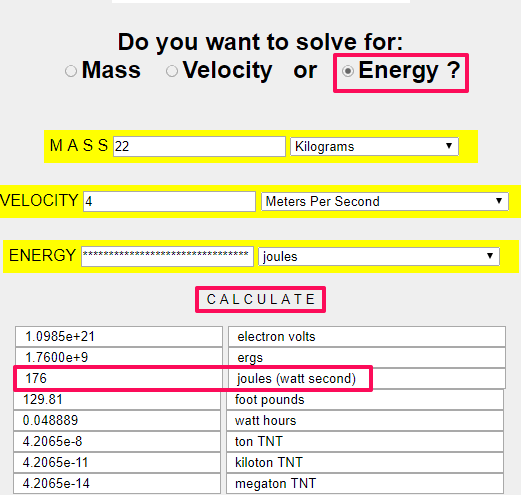Calculator City is a pretty nice Kinetic Energy Calculator website and you can also use it to carry out various other tasks. For calculating Kinetic Energy it provides a separate section. One of the best thing about this website is that it lets you calculate Kinetic Energy in different units. Also, you can choose various other units for the input parameters. Apart from standard units, it also supports, Grains, Grams, Ounces for mass, meters per second, kilometers per hour like units for velocity. And it lets you choose units for the final Kinetic Energy such as joules, ergs, electrons volts, watts hours, etc.

On the main interface of the website, it asks you whether you want to solve for mass, velocity or kinetic Energy. Select the Kinetic Energy option and after that specify the mass and velocity in the respective units you want. After that hit the Calculate button and it will calculate the Kinetic Energy for you in all available units. You can see it in the screenshot above.

## Kinetic Energy Calculator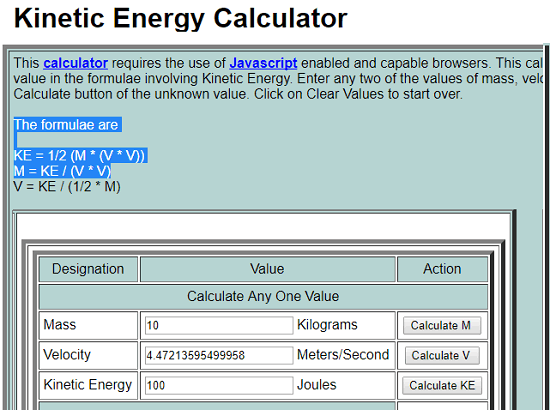Kinetic Energy Calculator (homepage) is another free Kinetic Energy Calculator website that you can use. Just specify the values for mass and velocity and then it will calculate the energy value for you. There are three fields for each Kinetic energy parameter. Specify any 2 parameters to find out third. The third parameter can be mass, velocity or Kinetic Energy. However, this website will not allow you to specify the units of the Kinetic Energy parameters. It will assume that you are dealing with the standard values.

## Convertalot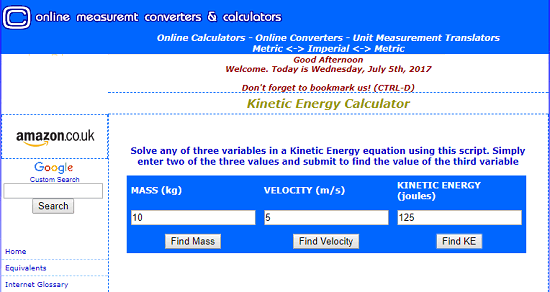Convertalot is another free and simple Kinetic Energy Calculator website. It lets you calculate Kinetic Energy after you specify mass and velocity magnitudes. Just like the previous website, you can also use it to find the third parameter with the help of any other two parameters. It uses the standard units to denote mass, velocity, and Kinetic energy. You can also use this website to do other Physics related conversion tasks, such as Length converter, Morse code translator, Hertz converter, heat index calculator and a lot of others.

The website has a pretty simple interface and you can easily get started with it. On its interface, you will see fields to specify values of mass, velocity, and Kinetic energy respectively. You can specify any two parameters and get the third one. To get Kinetic Energy, specify mass and velocity and hit the Find KE button. After that, it will show you the Kinetic Energy value which in Joules.

### Closing Words:

So, this is all about these free Kinetic Energy Calculator websites. Also, some of these websites let you calculate Kinetic Energy in various units. Personally, I really like Kinetic Energy Equations Formulas Calculator and Calculator City.

 Editor Ratings: User Ratings:[Total: 0 Average: 0]